# SSAT Upper Level Math : How to find the perimeter of a rectangle

## Example Questions

### Example Question #797 : Geometry

The length and width of a rectangle are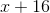and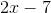. Give its perimeter in terms of.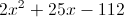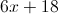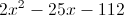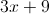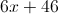Explanation:

A rectangle has perimeter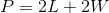the length and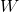the width. Substitute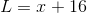and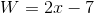in the perimeter formula, and simplify.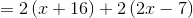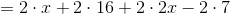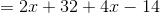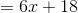### Example Question #1021 : Ssat Upper Level Quantitative (Math)

A rectangle has length 30 inches and width 25 inches. Which of the following is true about its perimeter?

Its perimeter is between 4 and 5 feet.

Its perimeter is between 10 and 11 feet.

Its perimeter is between 9 and 10 feet.

Its perimeter is between 8 and 9 feet.

Its perimeter is between 5 and 6 feet.

Its perimeter is between 9 and 10 feet.

Explanation:

In inches, the perimeter of the rectangle can be calculated by substituting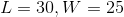in the following formula: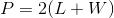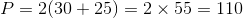The perimeter is 110 inches.

Now divide by 12 to convert to feet: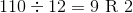This makes the perimeter 9 feet 2 inches, which is between 9 feet and 10 feet.

### Example Question #1 : How To Find The Perimeter Of A Rectangle

Perimeter of a rectangle is 36 inches. If the width of the rectangle is 3 inches less than its length, give the length and width of the rectangle.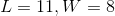inches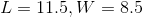inches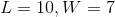inches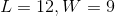inches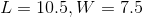inchesinches

Explanation:

Let: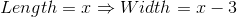The perimeter of a rectangle is, whereis the length andis the width of the rectangle. The perimeter is known so we can set up an equation in terms ofand solve it: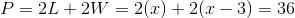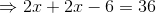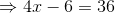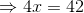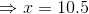So we can get: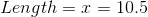inches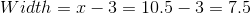inches

### Example Question #2 : How To Find The Perimeter Of A Rectangle

The length and width of a rectangle are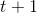and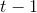, respectively. Give its perimeter in terms of.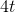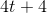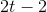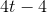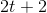Explanation:

The perimeter of a rectangle is, whereis the length andis the width of the rectangle. In order to find the perimeter we can substitute the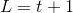and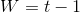in the perimeter formula: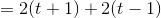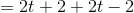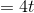### Example Question #3 : How To Find The Perimeter Of A Rectangle

The length of a rectangle is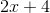and the width of this rectangle ismeters shorter than its length. Give its perimeter in terms of.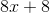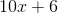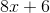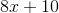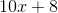Explanation:

The length of the rectangle is known, so we can find the width in terms of: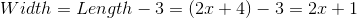The perimeter of a rectangle is, whereis the length andis the width of the rectangle.

In order to find the perimeter we can substitute the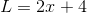and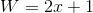in the perimeter formula: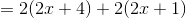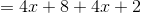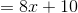### Example Question #4 : How To Find The Perimeter Of A Rectangle

A rectangle has a length of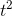inches and a width ofinches. Which of the following is true about the rectangle perimeter if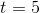?

Its perimeter is more than 8 feet.

Its perimeter is between 7.2 and 7.4 feet.

Its perimeter is between 7 and 8 feet.

Its perimeter is less than 7 feet.

Its perimeter is between 8 and 9 feet.

Its perimeter is between 7 and 8 feet.

Explanation:

Substitute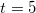to getand: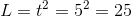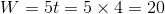The perimeter of a rectangle is, whereis the length andis the width of the rectangle. So we have: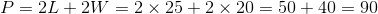inches

Now we should divide the perimeter by 12 in order to convert to feet: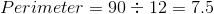feet

So the perimeter is 7 feet and 6 inches, which is between 7 and 8 feet.

### Example Question #1022 : Ssat Upper Level Quantitative (Math)

Which of these polygons has the same perimeter as a rectangle with length 55 inches and width 15 inches?

A regular pentagon with sidelength two feet

The other answer choices are incorrect.

A regular heptagon with sidelength two feet

A regular hexagon with sidelength two feet

A regular octagon with sidelength two feet

The other answer choices are incorrect.

Explanation:

The perimeter of a rectangle is twice the sum of its length and its width; a rectangle with dimensions 55 inches and 15 inches has perimeter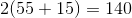inches.

All of the polygons in the choices are regular - that is, all have congruent sides - and all have sidelength two feet, or 24 inches, so we divide 140 by 24 to determine how many sides such a polygon would need to have a perimeter equal to the rectangle. However,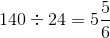,

so there cannot be a regular polygon with these characteristics. All of the choices fail, so the correct response is that none are correct.